PHY294H - Lecture 22

Ampere's law and Gauss' law

Last lecture we followed Ampere's orginal argument that the magnetic field near a long straight wire behaves as,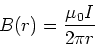(1)

The direction of the magnetic field is given by the right hand rule.

A more general form of this relation is,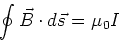(2)

I is the current which is enclosed within the closed path. This law enables us to choose the path of integration. If we can choose paths on which the magnetic field has simple behavior, then we can deduce the magnetic field in a very simple manner. This is similar to using Gauss' law in electrostatics. Note that there is also a Gauss' law for magnetic problems, so that the magnetic flux,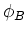, through a surface, S, is given by,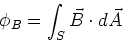(3)

which has the same form as the electric flux. However since there are no free magnetic charges, there is never any net enclosed magnetic charge inside a closed surface.

Example 30.1

Finding the magnetic field inside a current carrying wire. Consider a wire which has circular cross-section of radius R, and which carries current I which is uniformly distributed throughout the wire cross-section. Ampere's law is,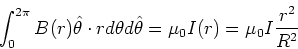(4)

From this we find,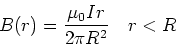(5)

For r>R, we have the usual relation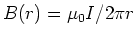.

Example - The solenoid

Take a loop which is a rectangle of length l and width b. If the solenoid is tightly packed, the magnetic field outside is zero. The magnetic fields at each end of the rectangular path should be the same, so on going around the loop, their contributions cancel. The remaining piece is the side which passes through the center of the solenoid. We thus have,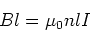(6)

where n is the number of turns per unit length. We thus have the simple relation.The thin toroid

We consider a solenoid which is formed into a circle, where the diameter of the solenoid is much smaller than the radius of the torus. We define a path through the center of the torus. On this path we expect the magnetic field to have the same magnitude, therefore,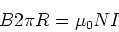(7)

So we have,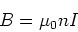(8)

as for the solenoid.

The Biot-Savart law

Ampere's law is great if we can find paths on which the magnetic field is constant or at least has a simple form. However this is not always possible. Consider for example a circular current loop. What magnetic field does it produce? To do calculations of this type, we need a new tool. The Biot-Savart law gives us this tool. The Biot-Savart law is a lot like the expression we used to calculate the electric field due to continuous charge distributions. In electrostatics, the electric field produced by a small element of charge dQ is given by,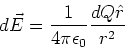(9)

The Biot-Savart law gives the magnetic field produced by a small element of current. The small element of current is usually written as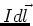, that is a constant current I flowing in a small length of wire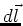. The magnetic field given by this small element of current is,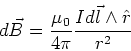(10)

The magnetic field is then found by integration, ie.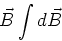(11)

Example - An infinite wire

Our first example is to find the magnetic field due to an infinite wire. Consider an infinite straight wire lying on the y-axis. We seek the magnetic field at a position x on the x-axis (i.e. at y=0). Consider a small element of current,centered at position -y on the y-axis. The distance from this element of current to position x on the x-axis is,

 R2 = x2+y2 (12)

The magnitude of the cross product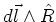is given by,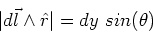(13)

where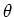is the angle between the vectors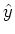and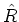. The direction of this cross product is perpendicular to bothandand so is in the z-direction for all values of y. That is, the only finite component of B is in the z-direction. Using the Biot-Savart law, we find the magnitude of the z-component of B to be,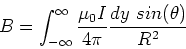(14)

From the geometry of the problem, we have,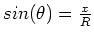, so we find,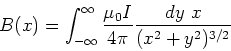(15)

Now we use the result,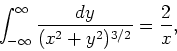(16)

so the final result is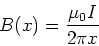(17)

Now note that the calculation above is the same for any choice of the x-axis, which means that our result is the same as that found by Ampere.